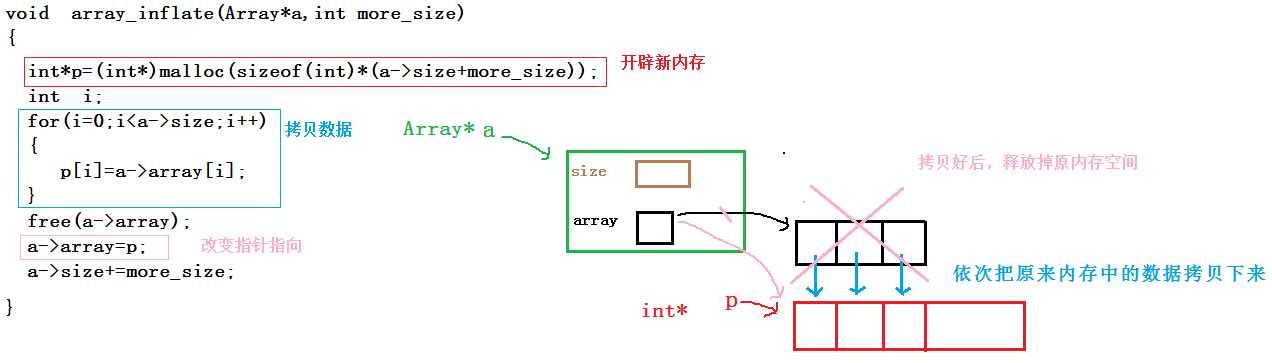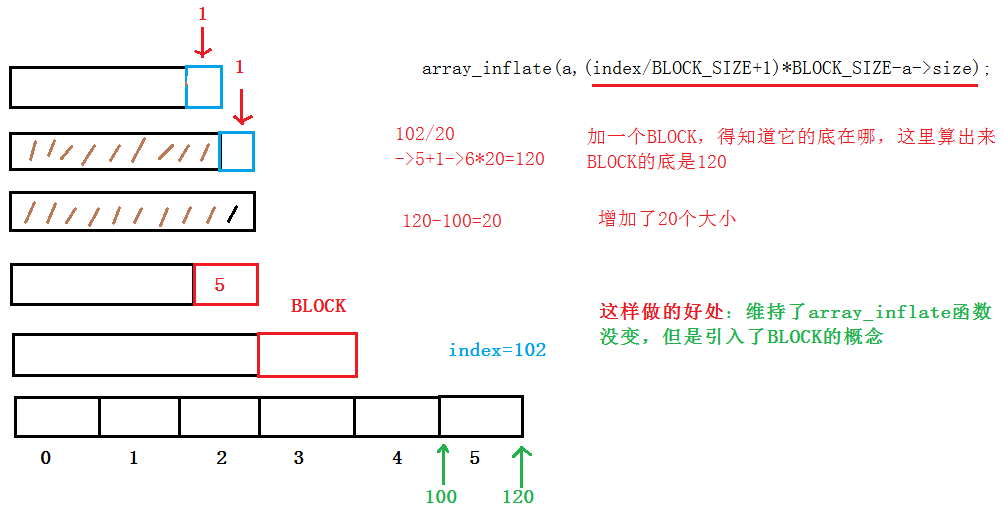# 用C语言简单实现一个可变数组

#ifndef _ARRAY_H_
#define _ARRAY_H_

const int BLOCK_SIZE = 2;
typedef struct
{
int*array;
int size;
}Array;

Array array_creat(int init_size);//创建一个数组
void array_free(Array *a);//回收空间
int  array_size(Array *a);//目前有多少个空间可以用
int*array_at(Array*a, int index);//访问数组当中某个单元:可以读也可以写（即可以做左值也可以做右值）
void array_inflate(Array*a, int more_size);//数组增容
void array_set(Array*a, int index, int value);//向数组中写入东西

#endif

array.cpp

#include"array.h"
#include<stdio.h>
#include<stdlib.h>

//创建一个数组
Array array_creat(int init_size)
{
Array a;
a.array = (int*)malloc(sizeof(int)*init_size);
a.size = init_size;
return a;
}

//回收空间
void array_free(Array *a)
{
free(a->array);
a->array = NULL;
a->size = 0;
}

//目前有多少个空间可以用
int  array_size(Array *a)
{
return a->size;
}

//访问数组当中某个单元:可以读也可以写（即可以做左值也可以做右值）
int*array_at(Array*a, int index)
{
if (index >= a->size)
{
array_inflate(a, (index / BLOCK_SIZE + 1)*BLOCK_SIZE - a->size);
}

return &(a->array[index]);
}

//数组增容
void array_inflate(Array*a, int more_size)
{
int*p = (int*)malloc(sizeof(int)*(a->size + more_size));//新开辟一段空间
int i;
//将原空间的内容全部拷贝至新空间
for (i = 0; i < a->size; i++)
{
p[i] = a->array[i];
}
//array_free(a);
free(a->array);
a->array = p;
a->size += more_size;

}
void array_set(Array*a, int index, int value)
{
a->array[index] = value;
}
int main()
{
Array a;
a=array_creat(3);
//array_free(&a);
*array_at(&a, 0) = 10;
*array_at(&a, 1) = 20;
*array_at(&a, 2) = 30;
*array_at(&a, 3) = 40;
*array_at(&a, 4) = 50;
*array_at(&a, 5) = 60;

//array_set(&a, 0, 10);
//array_set(&a, 1, 20);
//array_set(&a, 2, 30);

//array_inflate(&a, 2);

//array_set(&a, 3, 40);
//array_set(&a, 4, 50);
for (int i = 0; i < 4; i++)
{
printf("%d ", a.array[i]);
}
//printf("%d\n", array_size(&a));
array_free(&a);
//Array *pa= array_creat(NULL, 3);
//pa = array_creat(&a, 3);
//array_free(&a);
//array_free(&a);
system("pause");
return 0;
}

### 下面对代码的设计思路和重点部分讲解一下：

Array* array_creat(Array*a,int init_size)
{
a->size=init_size;
a->array=(int*)malloc(sizeof(int)*a->size);
return a;
}int*array_at(Array*a, int index)
{
if (index >= a->size)
{
array_inflate(a, index-a->size+1);//每次扩充一个大小
}
return &(a->array[index]);
}11-1829102-251万+
03-1621万+
07-04637
10-181万+
05-081万+
10-303万+
02-181214
05-221万+